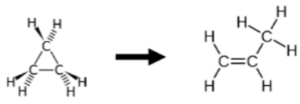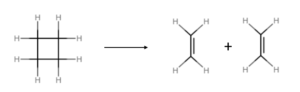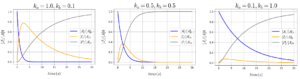# 17 Reaction Mechanisms – Elementary Reactions

Learning Objectives

By the end of this section, you should be able to:

Analyze elementary reactions and reaction molecularity

The sequence of events that occur at the molecular level during a reaction is the mechanism of the reaction. It describes how individual atoms, ions, or molecules interact to form particular products. The stepwise changes are collectively called the reaction mechanism. Each step of the reaction is called an elementary reaction. The sum of the elementary reactions in the mechanism must give the balanced chemical equation for the overall reaction. $^{}$

The decomposition of ozone, for example, appears to follow a mechanism with two steps:

$O_{3}→O_{2}+O$
$O+O_{3}→2O_{2}$

Elementary reactions add up to the overall reaction:

$2O_{3(g)}→3O_{2(g)}$

With the elementary reactions, we do not specify phases, and the formula represents specific processes occurring with individual molecules or atoms.

Notice that the oxygen atom produced in the first step of this mechanism is consumed in the second step and therefore does not appear as a product in the overall reaction. Species that are produced in one step and consumed in a subsequent step are called intermediates. $^{}$

## Molecularity

The molecularity is the number of molecules or atoms coming together to react in an elementary reaction.

• Unimolecular reaction – Only one molecule is involved, examples might include an isomerization or decomposition

Example: isomerization of cyclopropane to propeneExample: decomposition of cyclobutane• Bimolecular reaction – two molecules collide, interact, and undergo some kind of change. It can be two of the same or different molecules.

Example: Hydrogen attacking a bromine molecule

$H+Br_{2}→HBr+Br$

Reaction Order vs. Molecularity

Reaction order – An empirical quantity obtained from experimentally determined rate law

Molecularity – Number of molecules coming together in an elementary reaction proposed as an individual step in a mechanism

The order of the elementary reaction is the same as its molecularity. In contrast, the rate law cannot be determined from the balanced chemical equation for the overall reaction (unless it is a single step mechanism and is therefore also an elementary step).$^{}$

## Rate Laws for Elementary Reactions

### Unimolecular

$A→P$ (can be several products):

 $-\frac{d[A]}{dt}=k_{r}*[A]$

### Bimolecular

$A+B→P$ (can be several products):

 $-\frac{d[A]}{dt}=k_{r}*[A]*[B]$

$A+A→P$ (can be several products):

 $-\frac{d[A]}{dt}=k_{r}*[A]^2$

Generally these depend on concentration of reactants. However, these rate laws must be tested against experimental data.

## Consecutive Elementary Reactions

Some reactions proceed through the formation of an intermediate (I). One example of this with irreversible reactions is:

$A→I→P$

The intermediate($I$) is present in the reaction steps but does not appear in the overall reaction: $A→P$

Example: Express Rate of Formation for Reactive Compounds

Given the consecutive elementary reaction:

$A\xrightarrow{\text{ka}}I\xrightarrow{\text{kb}}P$

The rate of change for [A] is: $\frac{d[A]}{dt}=-k_{a}*[A]$

What are the equations for the rates of change for the other two species, $I$ and $P$, in terms of their concentrations and the reaction rate constants?

Solution

the intermediate I is produced by the reaction that converts A to I and consumed by the reaction that produces P:

$\frac{d[I]}{dt}=k_{a}*[A]-k_{b}*[I]$

P is produced by the reaction that converts I to P:

$\frac{d[P]}{dt}=k_{b}*[I]$

Taking 3 sets of arbitary values for $k_{a}$ and $k_{b}$, we can plot the following graphs of concentration vs.time:## References

 Chemistry LibreTexts. 2020. 14.6 Reaction Mechanisms.

 OpenStax Chemistry 2016. 12.6 Reaction Mechanisms. [online] Available at: <https://opentextbc.ca/chemistry/chapter/12-6-reaction-mechanisms> [Accessed 27 April, 2020].